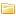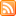# A soft Calculator that adds numbers together in a never-before-seen way

posted
updatedForum: Web, HTML, Tech

This is a program that adds numbers together in an unconventional way. If it were to ever be implemented at the hardware level, you would have much cheaper computers, and also lower power consumption.

https://github.com/36ddev/36ddev

This is a DOZENAL calculator, as opposed the normal decimal we are all used to. But it is peculiar, because it doesn't use zeros, and each end unit number, instead of "10" like we do for ten, it's just "d". That's right there are twelve number characters, and no zeros. Due to this, the number order counts 1-d (one thru twelve) in first row, then 21-2d (two-one thru two-twelve) for the second row. 31-3d for the third row, etc. So 13 (thirteen) in decimal is now written as 21 in this new way of doing things.

It works by grouping the numbers into 4 groups of threes. You add the threes together (thirds) and then add the groups together.

As an example:
Group1 Group2 Group3 Group4
1,2,3      1,2,3     1,2,3    1,2,3
1,2,3,     4,5,6,    7,8,9,    x,e,d

Let's do 5 + 2. 5 is a group 2 number. and it's the second "third" of group 2.
2 is a group 1 number, and it is also the second third but of group 1.
Let's do the math.

Thirds: 2+2 = 1  (the calculator overlaps after 3). So we know our third is a 1, somewhere.
Groups: 2 + 1 = 3. We get group 3...

So now we say, go to group 3, and drop down our third (1). 7 is the first third in group 3.
The answer is 7! Sounds easy right? But one thing, if your third sum is not OVER 3, then you do your group math (in this case 2+1 = 3) and then subtract a 1. So for instance, if we did 4 + 2:
Thirds: 1 + 2 = 3
Group: 2 + 1 = 3 (thirds not over 3) - 1 = 2.

Group 2, third 3.

It's a fun way of doing this, because it makes the associations between the numbers easier to understand. And again if implemented at t he hardware level would significantly simplify ALUs.

I plan to use this kind of logic in other programs to show the power of using this kind of ternary logic to do stuff. Well, what do you think

## 3 RepliesSort Replies:

posted

I wanted to bump this. I think this is really an important breakthrough in mathematics! At least for full adders in computers, it would make them so much cheaper to build using less electricity as well.